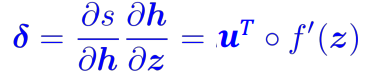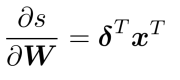# [XCS224N] Lecture 3 – Neural Networks

This week: neural net fundamentals

## Classification Setup and Notation

training data: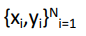### softmax classifier

(linear classifier — hyperplane):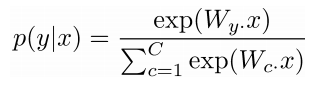ith row of the param W: weight vector for class i to compute logits: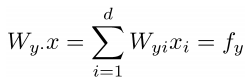prediction = softmax of f_y: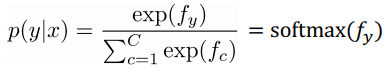### cross-entropy

goal: for (x, y), maximize p(y|x) ⇒ loss for (x, y) = -log p(y|x)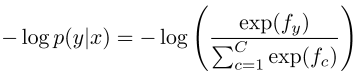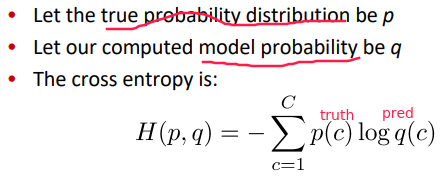in our case, the truth distribution is `one-hot` , i.e.p = [0, 0, ... , 1, ... 0] ⇒ cross entropy H = - sum{log q(y|x), for all x, y}

loss for all training data = averaging the losses: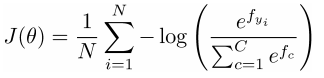where the logits vector `f` is: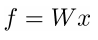## Neural Network Classifier

softmax or SVM or other linear models are not powerful enough ⇒ NN to learn nonlinear decision boundaries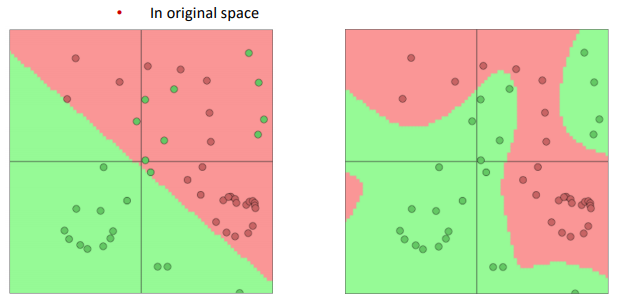in NLP: learn both model parameters ( `W` ) and representations (wordvecs `x` )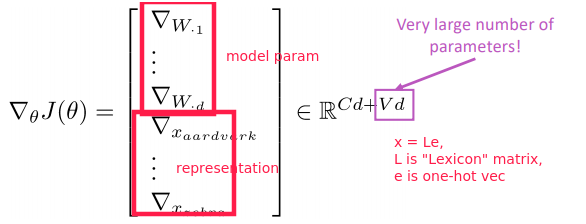artificial neuron: `y=f(Wx)` , where f is nonlinear activation func.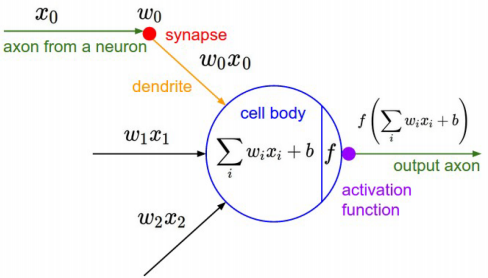when f = sigmoid = `1/(1+exp(-x))` , the neuron is binary logistic regression unit.

A neural network = running several logistic regressions at the same time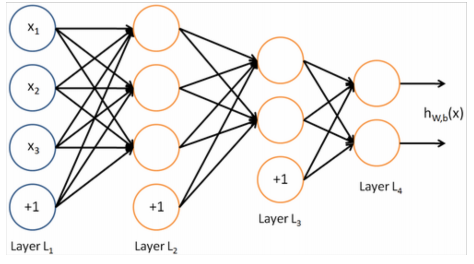matrix notation: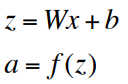Without non-linearities f(), deep neural networks can’t do anything more than a linear transform.

## Named Entity Recognition (NER)

task: find and classify names in text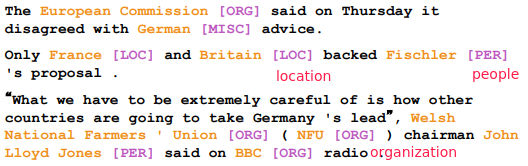BIO encoding: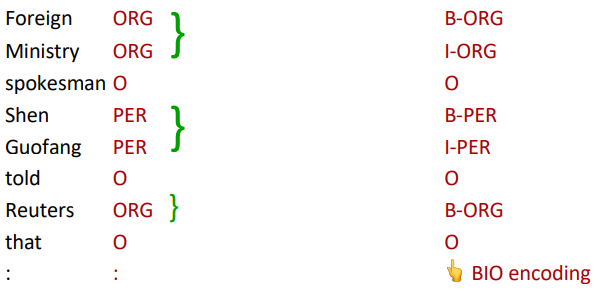## Binary Word Window Classification

Classify a word in its context window of neighboring words. simple idea: concat all context words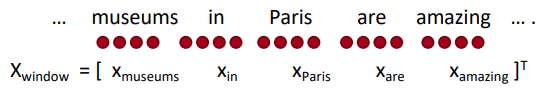Binary classification with unnormalized scores(2008&2011): build true window and corrupted windows.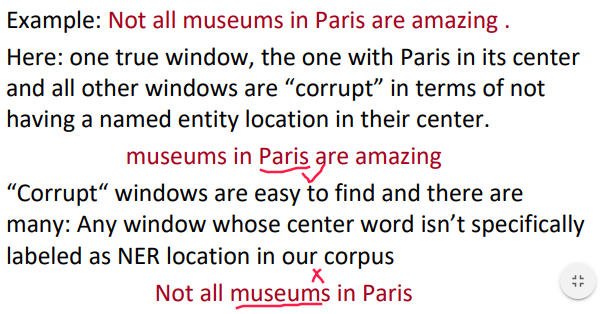feed-forward computation: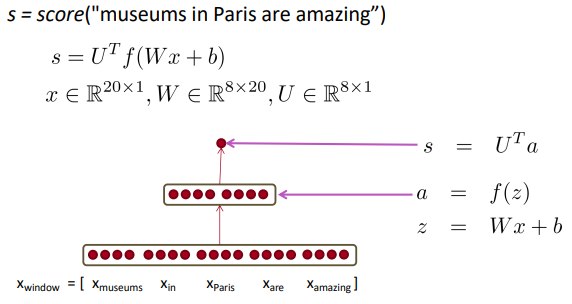intuition: middle layer learns non-linear interactions between words: example: only if “museums” is first vector should it matter that “in” is in the second position.

max-margin loss: let true window score be larger (by at leaset delta=1)than the corrupted window score.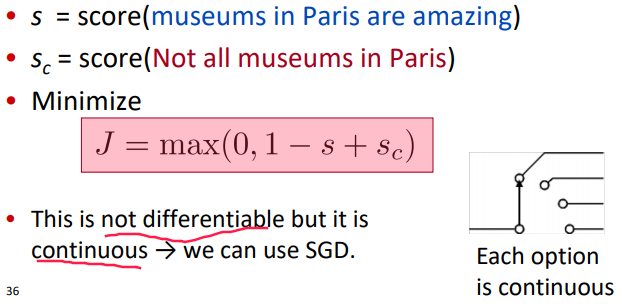QUESTION: why we can use SGD when continuous?

SGD: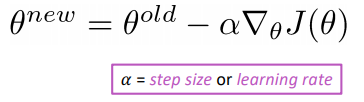multivariable derivatives / matrix calculus

• *when f is from Rn → R1, *Gradient=vector of partial derivatives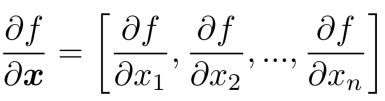• when f is from Rn → Rm, Jacobian is an m x n matrix of partial derivatives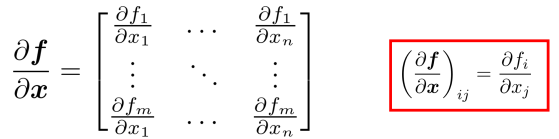chain rule: multiply the Jacobians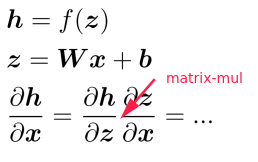⇒ the nonlinear (activation) function h is element-wise, Jacobian of h is diagonal: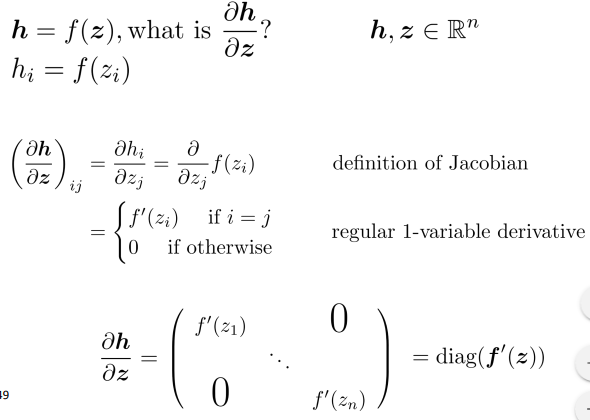⇒ other Jacobians: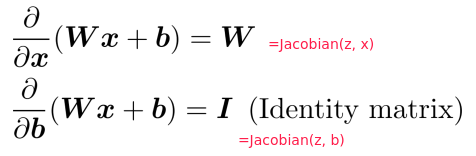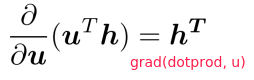notation: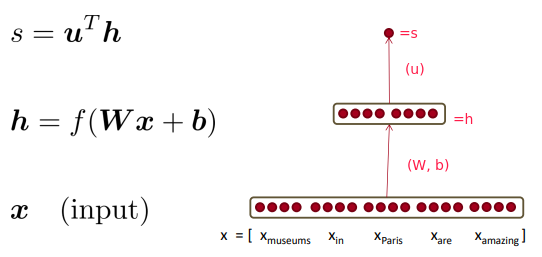⇒ Apply the chain rule with Jacobian/grad formulars from last section: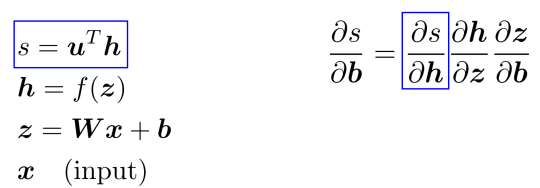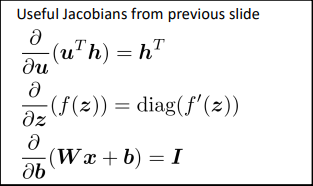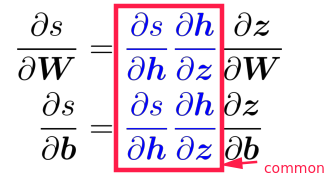⇒ extract the common part, call it local error signal: